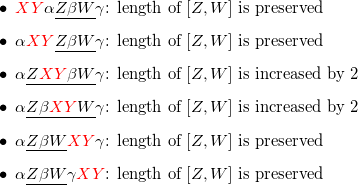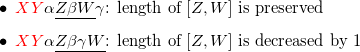# COUPLES - Editorial

Tester: Misha Chorniy
Editorialist: Suchan Park

Medium-Hard

### PREREQUISITES:

Sorting, Data structures, etc?

### PROBLEM:

There are N couples (consisting of two people) and 2N chairs along a circle. Each person is sitting on a chair.
What is the minimum number of adjacent swaps to make each couple sit next to each other?

### EXPLANATION:

Let’s assume chairs are numbered clockwise.

Consider each couple as an endpoint of a segment lying on a circle. Our goal is to make the length of each segment 1 by swapping adjacent endpoints.

## First, let’s focus on one particular segment.

Given two endpoints a and b, we can form two segments. Let’s define [a, b] as a segment on a circle from a to b clockwise. Therefore, [a, b] and [b, a] are different.

Suppose two endpoints X and Y are given. WLOG let X < Y. At the end, both endpoints should lie on [X, Y] or [Y, X] since they are adjacent.

If we only focus on this particular couple (WLOG, say X < Y), we can see that the number of swaps required to make them adjacent are:

• Y - X - 1 if both endpoints lie on [X, Y]
• 2N - (Y - X - 1) if both endpoints lie on [Y, X]

This is because the number of swaps equals to \texttt{distance}(X, X_i) + \texttt{distance}(Y, Y_i).

## However, there are lots of them

Unfortunately, there are N segments in total, and swapping adjacent elements do affect other segments. Let’s see what happens.

### What if we know all segments?

Suppose we already fixed which direction to choose ([a, b] or [b, a]), for all couples. There must exist some adjacent pair of chairs where no segment passes, because all circular permutations of N Ls and Rs, they all contain RL as a substring. Therefore we can break the circle into a line.

If two segments [X, Y] and [Z, W] don’t intersect, obviously they don’t affect each other. No explanation needed here.

Now, let’s consider the case when [X, Y] contains [Z, W]. The sequence can be written as “X \alpha Z \beta W \gamma Y” (where \alpha, \beta, \gamma are arbitrary sequences). Let’s see what happens after X and Y becomes adjacent by analyzing some possible cases:We can see the length of [Z, W] is preserved when X and Y becomes adjacent outside of the segment [Z, W], and increased by 2 otherwise. We already know that the number of required swaps increases when the segment length increases, so it seems we have to follow the former case.

Conversely, what if [X, Y] is contained by [Z, W]? X and Y will move inside the interval [Z, W], so the length of [Z, W] won’t change because of [X, Y]. Therefore we don’t need to consider this case.

The only remaining case is when [X, Y] and [Z, W] intersect but one doesn’t contain the other. WLOG, let’s write the sequence as “X \alpha Z \beta Y \gamma W”. Let’s see what happens after X and Y becomes adjacent by analyzing some possible cases:We can see the length of [Z, W] is decreased by 1 when X and Y becomes adjacent outside of the segment [Z, W], and increased by 1 if otherwise.

In summary,

• [X, Y] \cap [Z, W] = \varnothing or [X, Y] \in [Z, W]: doesn’t matter
• [Z, W] \in [X, Y]: XY should be adjacent outside of [Z, W] to preserve the interval length.
• [X, Y] \cap [Z, W] \neq \varnothing: XY should be adjacent outside of [Z, W] to decrease the interval length by 1.

Notice, that in both cases, if X < Z, just moving Y towards the right position of X doesn’t increase the length! (If X > Z, we have to move X towards the left position of Y)and this is the best we can do.

So, our strategy is simple: pick an non-adjacent segment which has the smallest left endpoint [X, Y], and move Y to the next of X. Continue this procedure until we can pick [X, Y]. In this way, the number of required swaps will be “sum of all segment lengths” subtracted by "the number of pairs of segments [a, b] and [c, d] such that a < c < b < d".

## But we don’t know which direction to choose

…but the above proof just assumes that we have N segments on a circle whose endpoints are all different. Therefore, we can just think of choosing one of [a, b] or [b, a] that has minimum length.

Wait, is it really optimal? Let’s see. Since the “sum of all segment lengths” are obviously minimum, we only need to see how "the number of pairs of segments [a, b] and [c, d] such that a < c < b < d" changes.

But, it turns out no matter what direction we choose, the number of such pairs doesn’t change!

Therefore this choice is obviously optimal.

## How to implement?

Suppose, for each 1 \le i \le N, we already computed l_i and r_i, the position of i inside A. (i.e. A[l_i] = A[r_i] = i)

Computing the sum of shorter lengths is just straightforward: \sum \min(r_i - l_i - 1, 2N - (r_i - l_i - 1)).

Computing the number of pairs of segments [a, b] and [c, d] such that a < c < b < d is not straightforward, since we need to compute where to split the circle, reordering the coordinates, … but wait! In the section above, we found out that no matter what direction we choose, the number of such pairs doesn’t change! Therefore, we just need to find the number of pairs i, j such that l_i < l_j < r_i < r_j, since it is just the same.

For subtask 1, just iterate all i and j and compute such pairs.

For subtask 2, we have to optimize this using data structures. Let’s assume that l_1 < l_2 < \cdots < l_N. For fixed j, we need to count the number of i < j (which implies l_i < l_j) such that l_j < r_i < r_j. Therefore, we can make an additional array temp which is initialized to 0, and run the algorithm below:

for(k = 1 to N) {
num_pairs = num_pairs + sum(temp[l_k .. r_k])
temp[r_k] = temp[r_k] + 1
}


Since we need to compute the range sum of temp and update one element of temp, we can use Fenwick trees or a segment tree.

### AUTHOR’S AND TESTER’S SOLUTIONS:

Author’s solution can be found here.
Tester’s solution can be found here.

1 Like

Author and tester solutions are not linked.